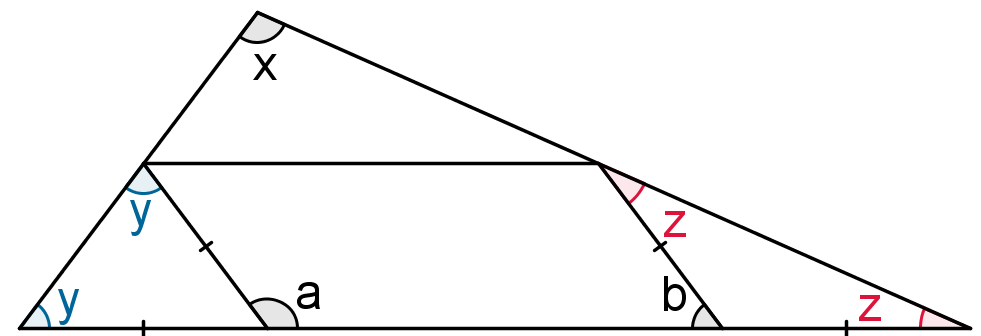#### You may also like### Getting an Angle

How can you make an angle of 60 degrees by folding a sheet of paper twice?### Arclets Explained

This article gives an wonderful insight into students working on the Arclets problem that first appeared in the Sept 2002 edition of the NRICH website.### Bow Tie

Show how this pentagonal tile can be used to tile the plane and describe the transformations which map this pentagon to its images in the tiling.

# Parallelogram in the Middle

##### Age 11 to 14 ShortChallenge LevelThe two angles marked $y ^{\circ}$ are equal because they are in an isosceles triangle. For the same reason, the angles $z^{\circ}$are equal. Since an exterior angle of a triangle is the sum of the two interior and opposite angles, it follows that $a=2y$ and $b=2z$. Now $a^{\circ}+ b^{\circ} = 180^{\circ}$ since they are the base angles of a parallelogram. So $2y + 2z = 180$ giving $y+z=90$. But, from the angle sum of a triangle $x+y+z=180$; hence $x =90$.
This problem is taken from the UKMT Mathematical Challenges.
You can find more short problems, arranged by curriculum topic, in our short problems collection.